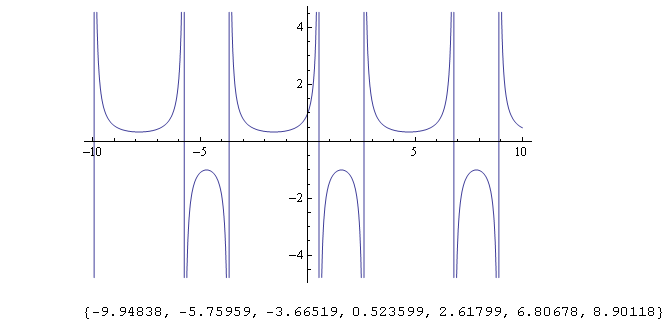# Can You Find The Points Of Discontinuity ,if Any A) F(x)=(1/(1-2sinx)) B) F(x)=(3/(5+2cosx))?

The points of discontinuity occur where the denominator goes to zero and changes sign.

A) 1-2sin[x] = 0
1 = 2sin[x]
1/2 = sin[x].
This will occur at x=(4n+1)Pi/2±Pi/3 radians = (4n+1)90±60 degrees, where n is any integer.  See the picture.

B) There are no points of discontinuity, because the minimum magnitude the denominator may have is 3. It never goes to zero.thanked the writer.